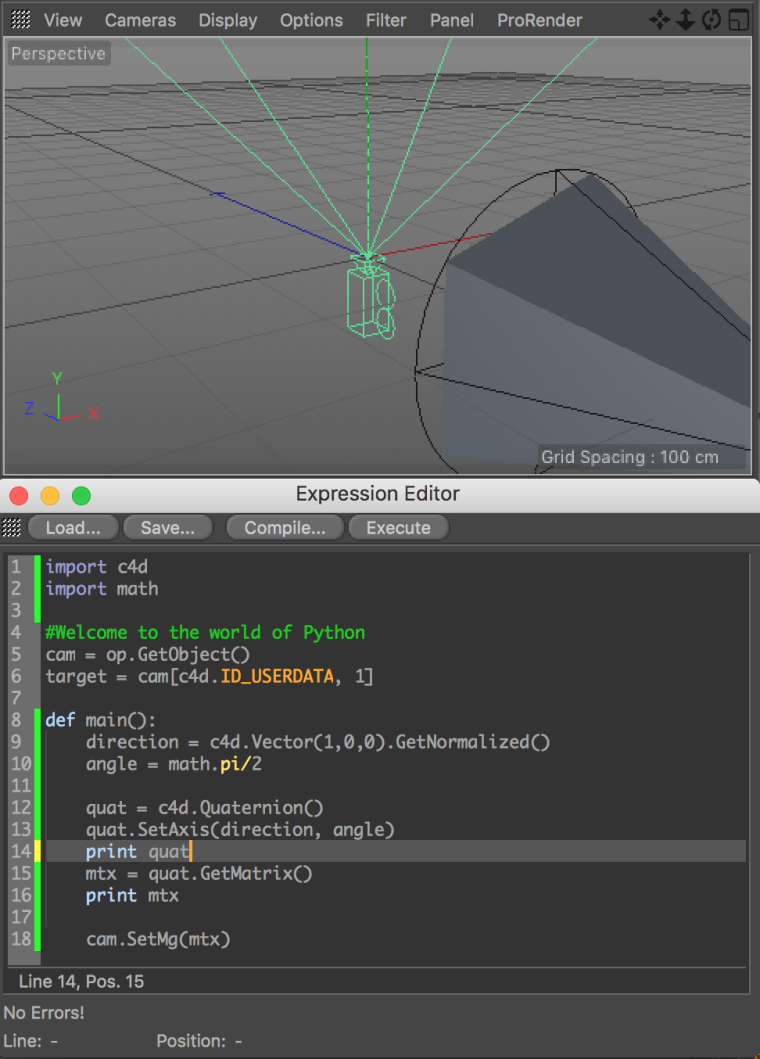# Solved Quaternion rotation

Hello friends,
I'm trying to work with the Quaternion-Object.
My cam-Object should align to the direction vector and pointed in this case in X-Direction.
But if print the q.v value I get a zero vector and the rotation is not applied
Does I misunderstood the Quaternion-Syntax? I can't find nothing about that.

Thanks

``````import c4d

cam = op.GetObject()

def main():
direction﻿ = c4d.Vector(10,0,0).GetNormalized()
angle = 0

q = c4d.Quaternion()
q.SetAxis(direction, angle)
b = q.GetMatrix()
b.off = cam.GetAbsPos()
cam.SetMg(b)

print q.v
``````

Hi,

Cheers,
Andreas

Hi Tudor,

actually your code is properly behaving, since the angle is set to 0. If, by chance, you set it to PI/2 you will immediately see that the camera promptly rotates and point up to the sky.

Hope the screenshot clarifies the situationBest, Riccardo

I thought I can use this direction vector as Quaternion-Axis. The cam should point than to the target.
But but it seems i need a different approach.

``````import c4d, math

cam = op.GetObject()
target = op[c4d.ID_USERDATA,1]

def main():
tarPos = target.GetAbsPos()
camPos = cam.GetAbsPos()

direction = (tarPos - camPos).GetNormalized()
angle = math.pi/2  #?

q = c4d.Quaternion()
q.SetAxis(direction, angle)
b = q.GetMatrix()
b.off = cam.GetAbsPos()
cam.SetMg(b)
``````

Hi Tudor, that's indeed not the way to proceed.

Quaternion is just a tool to conveniently represent a rotation in the space. That said the approach you need to follow is just to rotate the camera until it points where your target is.

Cheers, Riccardo

Hi,